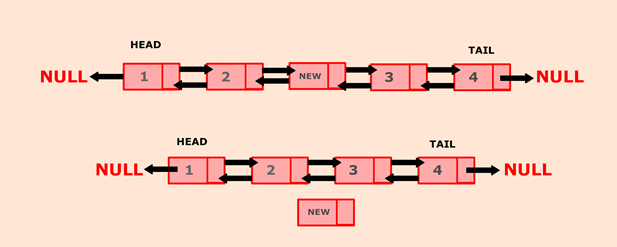Q:

# Java program to delete a new node from the middle of the doubly linked list

belongs to collection: Java Doubly Linked List Programs

In this program, we will create a doubly linked list and delete a node from the middle of the list. If the list is empty, display the message "List is empty". If the list is not empty, we will calculate the size of the list and then divide it by 2 to get the mid-point of the list. Current will point to head node. We will iterate through the list till mid?point is reached. Now current will point to the middle node. We delete middle node such that current's previous node will point to the current's next node.Consider the above example, mid-point of above list is 3. Iterate current from head to mid-point. Now, the current is pointing to mid node which needs to be deleted. In this case, node new is the middle node which needs to be deleted. New can be deleted by making node 2 (current's previous node) to point to node 3 (current's next node). Set current to null.

### Algorithm

• Define a Node class which represents a node in the list. It will have three properties: data, previous which will point to the previous node and next which will point to the next node.
• Define another class for creating the doubly linked list, and it has two nodes: head and tail. Initially, head and tail will point to null.
• deleteFromMid() will delete a node from the middle of the list:
• It first checks whether the head is null (empty list) then, it will return from the function as there is no node present in the list.
• If the list is not empty, it will check whether the list has only one node.
• If the list has more than one node then, it will calculate the size of the list. Divide the size by 2 and store it in variable mid.
• Iterate through the list till current points to mid node of the list.
• Connect current's previous node to current?s next node.
• Delete the current node by setting it to null.

a. display() will show all the nodes present in the list.

• Define a new node 'current' that will point to the head.
• Print current.data till current points to null.
• Current will point to the next node in the list in each iteration.

### Program:

``````class DeleteMid {

//Represent a node of the doubly linked list

class Node{
int data;
Node previous;
Node next;

public Node(int data) {
this.data = data;
}
}

public int size = 0;

//Create a new node
Node newNode = new Node(data);

//If list is empty
//Both head and tail will point to newNode
//head's previous will point to null
//tail's next will point to null, as it is the last node of the list
tail.next = null;
}
else {
//newNode will be added after tail such that tail's next will point to newNode
tail.next = newNode;
//newNode's previous will point to tail
newNode.previous = tail;
//newNode will become new tail
tail = newNode;
//As it is last node, tail's next will point to null
tail.next = null;
}
//Size will count the number of nodes present in the list
size++;
}

//deleteFromMid() will delete a node from middle of the list
public void deleteFromMid() {
//Checks whether list is empty
return;
}
else {

//Store the mid position of the list
int mid = (size % 2 == 0) ? (size/2) : ((size+1)/2);

//Iterate through list till current points to mid position
for(int i = 1; i < mid; i++){
current = current.next;
}

//If middle node is head of the list
}
//If middle node is tail of the list
else if(current == tail) {
tail = tail.previous;
}
else {
current.previous.next = current.next;
current.next.previous = current.previous;
}
//Delete the middle node
current = null;
}
size--;
}

//display() will print out the nodes of the list
public void display() {
//Node current will point to head
System.out.println("List is empty");
return;
}
while(current != null) {
//Prints each node by incrementing the pointer.

System.out.print(current.data + " ");
current = current.next;
}
System.out.println();
}

public static void main(String[] args) {

DeleteMid dList = new DeleteMid();

//Printing original list
System.out.println("Original List: ");
dList.display();
dList.deleteFromMid();
//Printing updated list
System.out.println("Updated List: ");
dList.display();
}
}
}  ``````

Output:

```Original List:
1 2 3 4 5
Updated List:
1 2 4 5
Updated List:
1 4 5
Updated List:
1 5
Updated List:
5
Updated List:
List is empty```